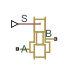# Orifice with Variable Area Slot

(To be removed) Hydraulic variable orifice shaped as rectangular slot

The Hydraulics (Isothermal) library will be removed in a future release. Use the Isothermal Liquid library instead. (since R2020a)

## Library

Orifices

•## Description

The block models a variable orifice created by a cylindrical sharp-edged spool and a rectangular slot in a sleeve. The flow rate through the orifice is proportional to the orifice opening and to the pressure differential across the orifice. The flow rate is determined according to the following equations:

`$q={C}_{D}\cdot A\left(h\right)\sqrt{\frac{2}{\rho }}\frac{\Delta p}{{\left(\Delta {p}^{2}+{p}_{\text{Cr}}^{2}\right)}^{1/4}},$`
`$\Delta p={p}_{\text{A}}-{p}_{\text{B}},$`
`$h={x}_{0}+x·or$`

where

 q Flow rate p Pressure differential pA, pB Gauge pressures at the block terminals CD Flow discharge coefficient A(h) Instantaneous orifice passage area b Width of the orifice slot x0 Initial opening x Spool displacement from initial position h Orifice opening or Orifice orientation indicator. The variable assumes +1 value if a spool displacement in the globally assigned positive direction opens the orifice, and –1 if positive motion decreases the opening. ρ Fluid density Aleak Closed orifice leakage area pcr Minimum pressure for turbulent flow

The minimum pressure for turbulent flow, pcr, is calculated according to the laminar transition specification method:

• By pressure ratio — The transition from laminar to turbulent regime is defined by the following equations:

pcr = (pavg + patm)(1 – Blam)

pavg = (pA + pB)/2

where

 pavg Average pressure between the block terminals patm Atmospheric pressure, 101325 Pa Blam Pressure ratio at the transition between laminar and turbulent regimes (Laminar flow pressure ratio parameter value)
• By Reynolds number — The transition from laminar to turbulent regime is defined by the following equations:

`${p}_{cr}=\frac{\rho }{2}{\left(\frac{{\mathrm{Re}}_{cr}\cdot \nu }{{C}_{D}\cdot {D}_{H}}\right)}^{2}$`
`${D}_{H}=\sqrt{\frac{4A}{\pi }}$`

where

 DH Instantaneous orifice hydraulic diameter ν Fluid kinematic viscosity Recr Critical Reynolds number (Critical Reynolds number parameter value)

The block positive direction is from port A to port B. This means that the flow rate is positive if it flows from A to B and the pressure differential is determined as $\Delta p={p}_{\text{A}}-{p}_{\text{B}},$. Positive signal at the physical signal port `S` opens or closes the orifice depending on the value of the parameter Orifice orientation.

## Basic Assumptions and Limitations

• Fluid inertia is not taken into account.

## Parameters

Orifice width

The width of the rectangular slot. The default value is `1e-2` m.

Flow discharge coefficient

Semi-empirical parameter for orifice capacity characterization. Its value depends on the geometrical properties of the orifice, and usually is provided in textbooks or manufacturer data sheets. The default value is `0.7`.

Initial opening

Orifice initial opening. The parameter can be positive (underlapped orifice), negative (overlapped orifice), or equal to zero for zero lap configuration. The value of initial opening does not depend on the orifice orientation. The default value is `0`.

Orifice orientation

The parameter is introduced to specify the effect of the orifice control member motion on the valve opening. The parameter can be set to one of two options: `Opens in positive direction` or ```Opens in negative direction```. The value ```Opens in positive direction``` specifies an orifice whose control member opens the valve when it is shifted in the globally assigned positive direction. The parameter is extremely useful for building a multi-orifice valve with all the orifices being controlled by the same spool. The default value is `Opens in positive direction`.

Laminar transition specification

Select how the block transitions between the laminar and turbulent regimes:

• `Pressure ratio` — The transition from laminar to turbulent regime is smooth and depends on the value of the Laminar flow pressure ratio parameter. This method provides better simulation robustness.

• `Reynolds number` — The transition from laminar to turbulent regime is assumed to take place when the Reynolds number reaches the value specified by the Critical Reynolds number parameter.

Laminar flow pressure ratio

Pressure ratio at which the flow transitions between laminar and turbulent regimes. The default value is `0.999`. This parameter is visible only if the Laminar transition specification parameter is set to `Pressure ratio`.

Critical Reynolds number

The maximum Reynolds number for laminar flow. The value of the parameter depends on the orifice geometrical profile. You can find recommendations on the parameter value in hydraulics textbooks. The default value is `12`. This parameter is visible only if the Laminar transition specification parameter is set to `Reynolds number`.

Leakage area

The total area of possible leaks in the completely closed valve. The main purpose of the parameter is to maintain numerical integrity of the circuit by preventing a portion of the system from getting isolated after the valve is completely closed. The parameter value must be greater than 0. The default value is `1e-12` m^2.

## Global Parameters

Parameters determined by the type of working fluid:

• Fluid density

• Fluid kinematic viscosity

Use the Hydraulic Fluid block or the Custom Hydraulic Fluid block to specify the fluid properties.

## Ports

The block has the following ports:

`A`

Hydraulic conserving port associated with the orifice inlet.

`B`

Hydraulic conserving port associated with the orifice outlet.

`S`

Physical signal port to control spool displacement.

The flow rate is positive if fluid flows from port `A` to port `B`. Positive signal at the physical signal port `S` opens or closes the orifice depending on the value of the parameter Orifice orientation.

## Version History

Introduced in R2006a

expand all